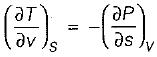Courses

# Test: Thermodynamic Relations - 3

## 10 Questions MCQ Test Thermodynamics | Test: Thermodynamic Relations - 3

Description
This mock test of Test: Thermodynamic Relations - 3 for Mechanical Engineering helps you for every Mechanical Engineering entrance exam. This contains 10 Multiple Choice Questions for Mechanical Engineering Test: Thermodynamic Relations - 3 (mcq) to study with solutions a complete question bank. The solved questions answers in this Test: Thermodynamic Relations - 3 quiz give you a good mix of easy questions and tough questions. Mechanical Engineering students definitely take this Test: Thermodynamic Relations - 3 exercise for a better result in the exam. You can find other Test: Thermodynamic Relations - 3 extra questions, long questions & short questions for Mechanical Engineering on EduRev as well by searching above.
QUESTION: 1

### Match List-I with List-ll and select the correct answer using the codes given below the lists: List-I A. Volume expansivity (β) B. Joule-kelvin coefficient (μJ) C. Adiabatic compressibility (KS) List-ll 1.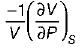2.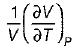3.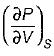4.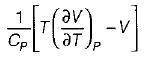Codes:       A  B C (a)  3  4  1 (b)  2  4  1 (c)  3  1  4 (d)  2  1  4

Solution:

Volume expansivity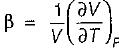Joule thomson coefficient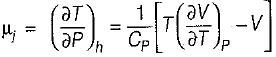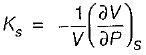QUESTION: 2

### When a real gas undergoes Joule-thomson expansion the temperature

Solution: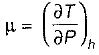If μ is positive ⇒ temperature decreases during throttling
If μ is negative ⇒ Temperature increases during throttling.

QUESTION: 3

### The specific heat relation is

Solution: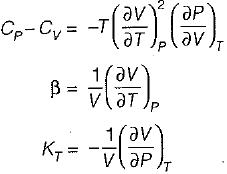we get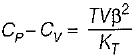QUESTION: 4

TdS equation is

Solution:
QUESTION: 5

It can be shown that for simple compressible substance, the relationship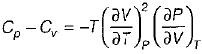exists where, Cp & Cv are specific heats at constant pressure and constant volume respectively. T is temperature, Vis volume and Pis pressure. Which one of the following statements is NOT true?

Solution: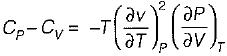Since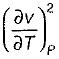is always positive and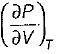for any substance is negative (C- Cv) Is always positive. Therefore Cp is always greater then Cv. When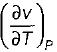= 0 (for water at 4°C when density is maximum or specific volume minimum), Cp - Cv

QUESTION: 6

For a given volume of dry saturated steam Clapeyron’s equation is given by

Solution:
QUESTION: 7

For a single component two-phase mixture the number of independent variable properties are

Solution:

From the Gibb’s phase rule
C + 2 = P + F
C = 1 (Single component)
P = 2 (Two-phase)
F = C + 2 - P - 1 + 2 - 2
F = 1

QUESTION: 8

On a P - V diagram of an ideal gas, suppose a reversible a diabatic line intersects a reversible isothermal line at point A.Then at point A, the slope of the reversible adiabatic line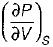and the slope of the reversible isothermal line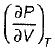are related as

Solution: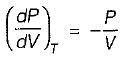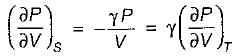QUESTION: 9

Which of the following identities can be most easily used to verify steam table data for superheated steam

Solution:
QUESTION: 10

For a pure substance, the Maxwell’s reiatior obtained from the fundamental property relation du = Ids - Pdv is

Solution:

Given,
dU = Tds - Pdv
This is an exact differential equation, so